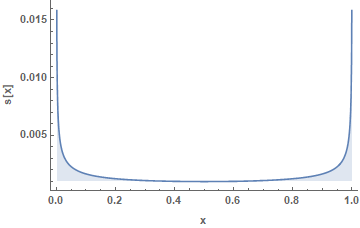So, I was working on a regression problem and my $$y$$ values, in theory, would fall in the range $$[0,1]$$. In reality, though, most of them were crowded between $$0.9$$ and $$1.0$$. I thought that I could apply some transformation and distribute them more evenly, without thinking about it too much.

Therefore, I applied the arc sine square transformation, again, without checking when this transformation would make sense.

$z = \text{arcsin}(\sqrt{y})$

After running my code to the transformed data set, I noticed that not only did the model not perform better, but the results I was getting were very bad.

The reason behind this failure is, I guess, that my data were noisy and this transformation inflated the error. You can check this wikipedia article on propagation of uncertainty. A common formula to calculate error propagation is the following. Assuming that you have $$z = f(x, y, \ldots)$$, then your error in the variable $$z$$ is given by:

$s_z = \sqrt{ \left(\frac{\partial f}{\partial x}\right)^2 s_x^2 + \left(\frac{\partial f}{\partial y} \right)^2 s_y^2 + \cdots}$

Where $$s_z$$ represents the standard deviation of the function $$f$$, $$s_x$$ represents the standard deviation of $$x$$, $$s_y$$ represents the standard deviation of $$y$$ and so forth.

So, in my case it was simply this:

$s_z = \frac{\mathrm{d} z}{\mathrm{d} y} s_y \Rightarrow s_z = \frac{1}{2\sqrt{y (1-y)}} s_y$

And since my $$y$$’s were very close to $$1$$, naturally $$s_z$$ exploded.Moral: don’t try random stuff; make educated guesses.

Excercise for the reader: What data transformation would actually reduce my error? Can you think of some function $$z = f(y)$$, such as that when you calculate $$\frac{\mathrm{d} z}{\mathrm{d} y}$$, $$s_z$$ is smaller compared to $$s_y$$ for $$y$$ values close to $$1$$?